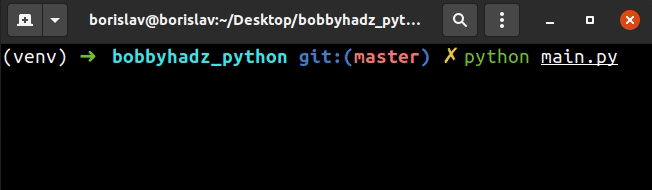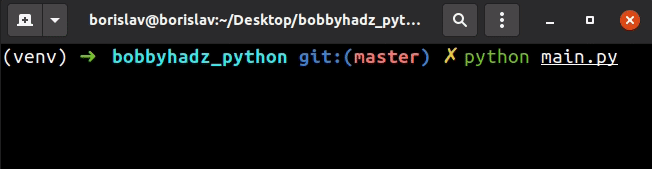# Create a date from user Input in PythonLast updated: Aug 25, 2022Photo from Unsplash

## Create a date from user Input in Python#

To create a date from user input:

1. Use the `input()` function to take input from the user.
2. Take values for the year, month and day of the month.
3. Use the `date()` class from the `datetime` module to create the date.
main.py
```Copied!```from datetime import date, datetime

year = int(input('Enter a year: '))
month = int(input('Enter a month: '))
day = int(input('Enter a day: '))

d = date(year, month, day)
print(d)

# ---------------------------------------

# 👇️ date and time

hours = int(input('Enter the hour: '))
minutes = int(input('Enter the minutes: '))
seconds = int(input('Enter the seconds: '))

dt = datetime(year, month, day, hours, minutes, seconds)

print(dt)
``````We used the `input()` function to take input from the user.

The input function takes an optional `prompt` argument and writes it to standard output without a trailing newline.

The function then reads the line from input, converts it to a string and returns the result.

Note that the `input()` function is guaranteed to return a string even if the user enters a number.

This is why we had to use the `int()` class to convert the values before passing them to the `date()` and `datetime()` classes.

main.py
```Copied!```from datetime import date

year = int(input('Enter a year: '))
month = int(input('Enter a month: '))
day = int(input('Enter a day: '))

d = date(year, month, day)
print(d)
``````

If you need to create a `datetime` object, take values for the hours, minutes and seconds as well.

main.py
```Copied!```from datetime import datetime

year = int(input('Enter a year: '))
month = int(input('Enter a month: '))
day = int(input('Enter a day: '))

hours = int(input('Enter the hour: '))
minutes = int(input('Enter the minutes: '))
seconds = int(input('Enter the seconds: '))

dt = datetime(year, month, day, hours, minutes, seconds)

print(dt)
``````

Alternatively, you can take a single input value from the user and split it into date components.

To create a date from user input:

1. Use the `input()` function to take a value formatted as `YYYY-MM-DD`.
2. Split the input value on the hyphens and convert the date components to integers.
3. Use the `date()` class from the `datetime` module to create the date.
main.py
```Copied!```from datetime import date

date_components = input('Enter a date formatted as YYYY-MM-DD: ').split('-')
print(date_components)

year, month, day = [int(item) for item in date_components]

d = date(year, month, day)

print(d)
``````We use the `input()` function to take a string formatted as `YYYY-MM-DD` and use the `str.split()` method to split it on each hyphen.

The str.split() method splits the string into a list of substrings using a delimiter.

main.py
```Copied!```print('2023-11-22'.split('-')) # 👉️ ['2023', '11', '22']

print('2024-06-21'.split('-')) # 👉️ ['2024', '06', '21']
``````

The method takes the following 2 parameters:

NameDescription
separatorSplit the string into substrings on each occurrence of the separator
maxsplitAt most `maxsplit` splits are done (optional)

The next step is to use a list comprehension to convert the strings to integers.

main.py
```Copied!```from datetime import date

date_components = input('Enter a date formatted as YYYY-MM-DD: ').split('-')
print(date_components)

year, month, day = [int(item) for item in date_components]

d = date(year, month, day)

print(d)
``````
List comprehensions are used to perform some operation for every element or select a subset of elements that meet a condition.

On each iteration, we use the `int()` class to convert the current string to an integer and return the result.

The last step is to pass the date components to the `date` class to create a date object.

I wrote a book in which I share everything I know about how to become a better, more efficient programmer.You can use the search field on my Home Page to filter through all of my articles.